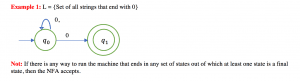## Example of Conversion of Non-Deterministic Finite Automata (NFA) to Deterministic Finite Automata (DFA) – 2

Example :  L = {Set of all string over (0,1) that ends with 1}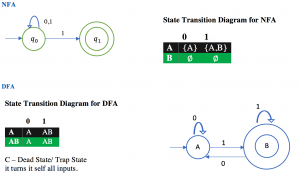## Example of Conversion of Non-Deterministic Finite Automata (NFA) to Deterministic Finite Automata (DFA) – 1

Example:  L= {Set of all strings over (0,1) that start with 0}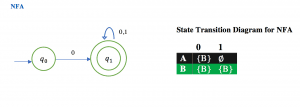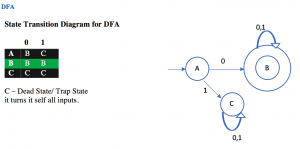## Example of Non-Deterministic Finite Automata (NFA) – 8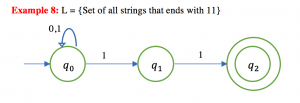## Example of Non-Deterministic Finite Automata (NFA) – 7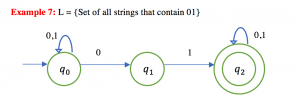## Example of Non-Deterministic Finite Automata (NFA) – 6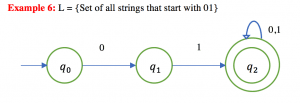## Example of Non-Deterministic Finite Automata (NFA) – 5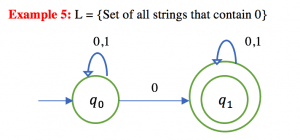## Example of Non-Deterministic Finite Automata (NFA) – 4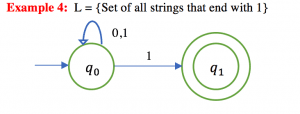## Example of Non-Deterministic Finite Automata (NFA) – 3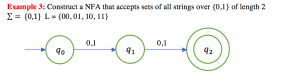## Example of Non-Deterministic Finite Automata (NFA) – 2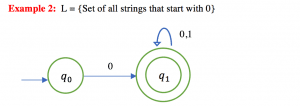## Example of Non-Deterministic Finite Automata (NFA) – 1